/

### Function Description

Returns the de-smoothing parameter corresponding to the AR(1) de-smoothed (or ‘de-correlated) values of a series, as described in the book Extreme Events. This involves:

(a)    Postulating (for, say, a return series) that there is some underlying ‘true’ return series,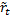, and that the observed series,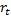, derives from it via a first order autoregressive model,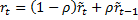; and

(b)   Identifying the value of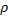(between 0 and 0.5) for whichhas zero first order correlation (which implies that the covariance between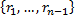and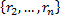is zero.

The de-smoothed series,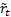, can then be derived using the following formula, see MnDesmooth_AR1: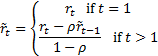Contents | Prev | Next